# Tsovaltzi etal ectel2010

7. Oct 2010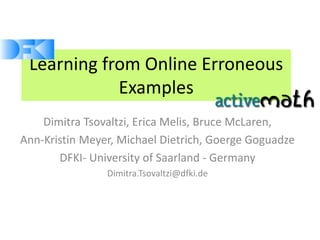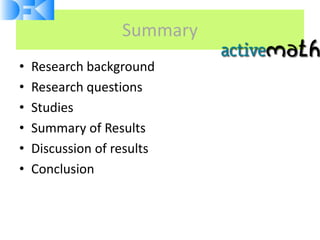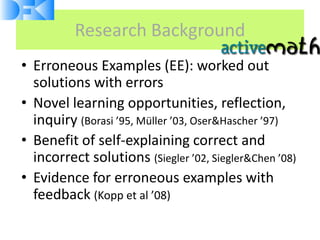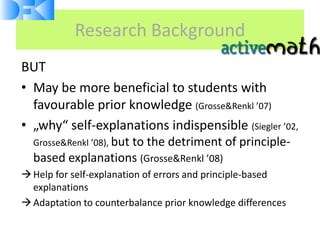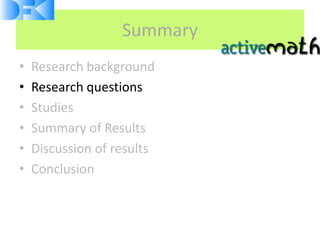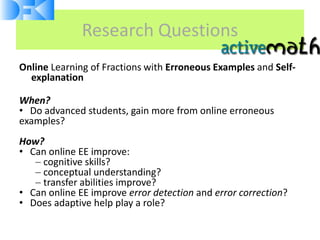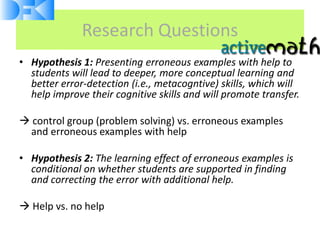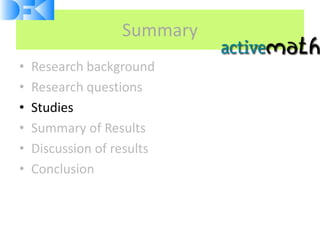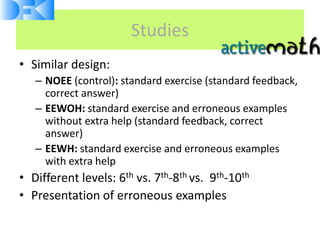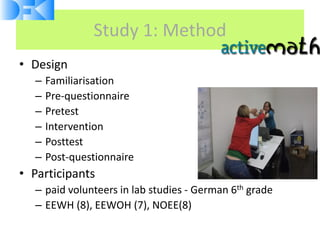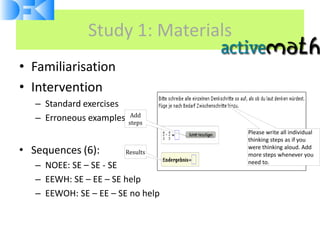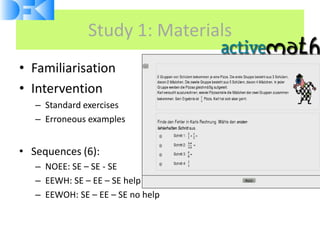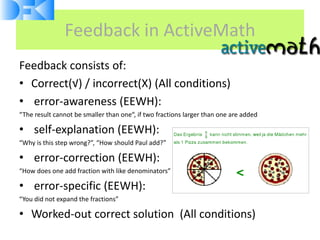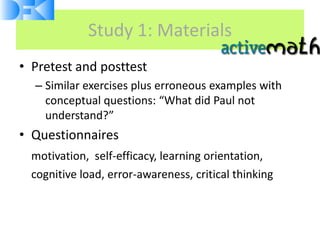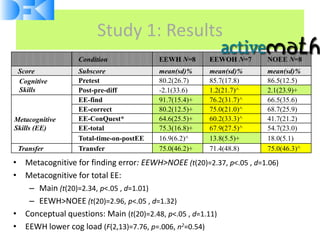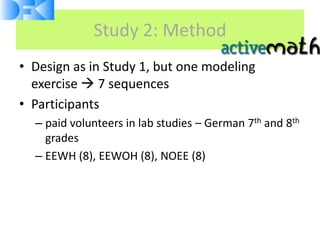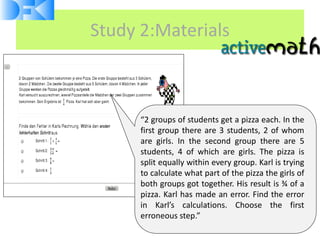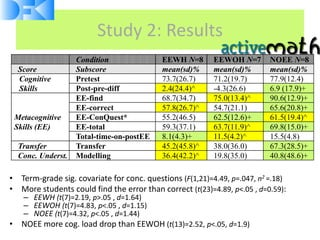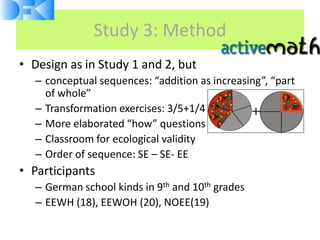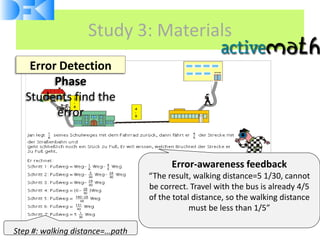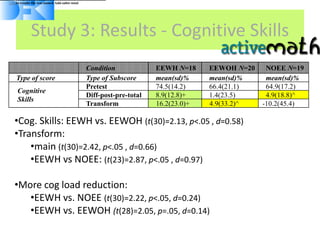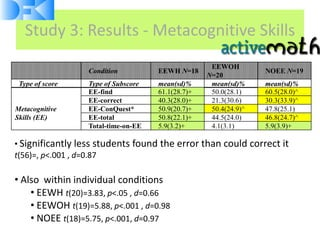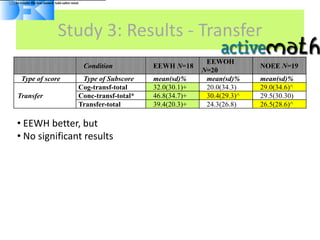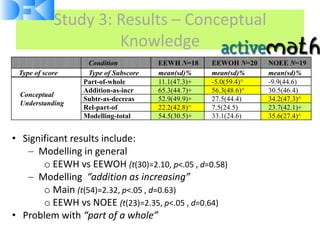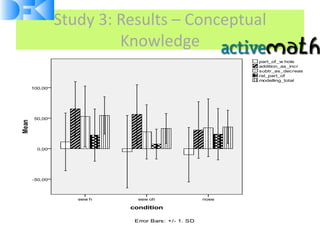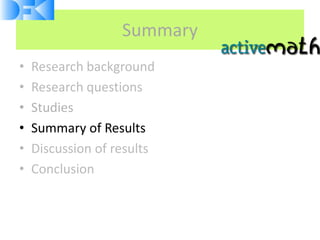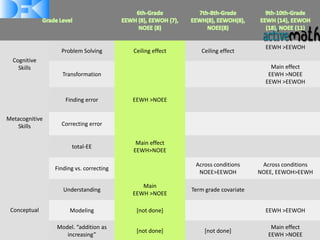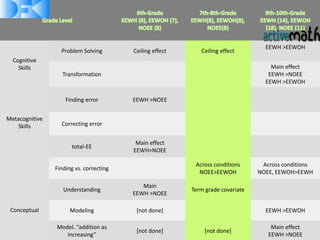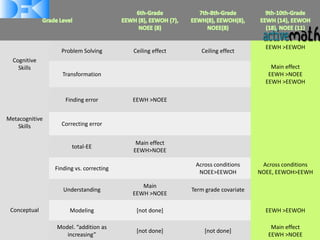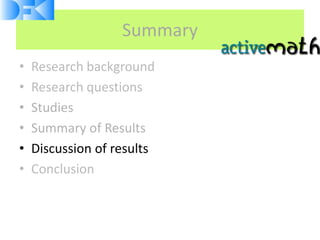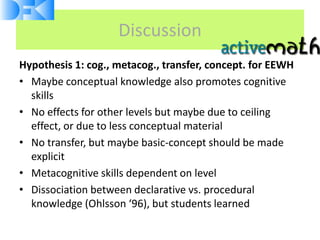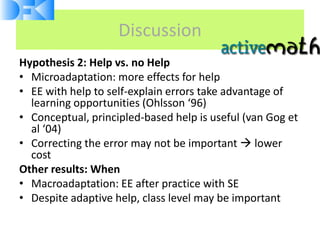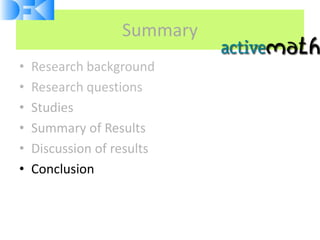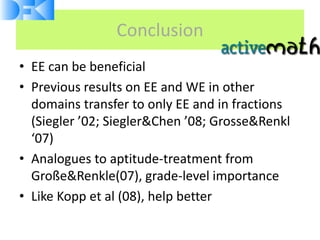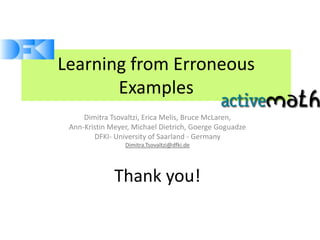1 von 36

### Tsovaltzi etal ectel2010

• 1. Learning from Online Erroneous Examples Dimitra Tsovaltzi, Erica Melis, Bruce McLaren, Ann-Kristin Meyer, Michael Dietrich, Goerge Goguadze DFKI- University of Saarland - Germany Dimitra.Tsovaltzi@dfki.de
• 2. Summary • Research background • Research questions • Studies • Summary of Results • Discussion of results • Conclusion
• 3. Research Background • Erroneous Examples (EE): worked out solutions with errors • Novel learning opportunities, reflection, inquiry (Borasi ’95, Müller ’03, Oser&Hascher ’97) • Benefit of self-explaining correct and incorrect solutions (Siegler ’02, Siegler&Chen ’08) • Evidence for erroneous examples with feedback (Kopp et al ’08)
• 4. Research Background BUT • May be more beneficial to students with favourable prior knowledge (Grosse&Renkl ’07) • „why“ self-explanations indispensible (Siegler ’02, Grosse&Renkl ’08), but to the detriment of principle- based explanations (Grosse&Renkl ’08)  Help for self-explanation of errors and principle-based explanations  Adaptation to counterbalance prior knowledge differences
• 5. Summary • Research background • Research questions • Studies • Summary of Results • Discussion of results • Conclusion
• 6. Research Questions Online Learning of Fractions with Erroneous Examples and Self- explanation When? • Do advanced students, gain more from online erroneous examples? How? • Can online EE improve: cognitive skills? conceptual understanding? transfer abilities improve? • Can online EE improve error detection and error correction? • Does adaptive help play a role?
• 7. Research Questions • Hypothesis 1: Presenting erroneous examples with help to students will lead to deeper, more conceptual learning and better error-detection (i.e., metacogntive) skills, which will help improve their cognitive skills and will promote transfer.  control group (problem solving) vs. erroneous examples and erroneous examples with help • Hypothesis 2: The learning effect of erroneous examples is conditional on whether students are supported in finding and correcting the error with additional help.  Help vs. no help
• 8. Summary • Research background • Research questions • Studies • Summary of Results • Discussion of results • Conclusion
• 9. Studies • Similar design: – NOEE (control): standard exercise (standard feedback, correct answer) – EEWOH: standard exercise and erroneous examples without extra help (standard feedback, correct answer) – EEWH: standard exercise and erroneous examples with extra help • Different levels: 6th vs. 7th-8th vs. 9th-10th • Presentation of erroneous examples
• 10. Study 1: Method • Design – Familiarisation – Pre-questionnaire – Pretest – Intervention – Posttest – Post-questionnaire • Participants – paid volunteers in lab studies - German 6th grade – EEWH (8), EEWOH (7), NOEE(8)
• 11. Study 1: Materials • Familiarisation • Intervention – Standard exercises Add – Erroneous examples steps Please write all individual thinking steps as if you • Sequences (6): Results were thinking aloud. Add more steps whenever you – NOEE: SE – SE - SE need to. – EEWH: SE – EE – SE help – EEWOH: SE – EE – SE no help
• 12. Study 1: Materials • Familiarisation • Intervention – Standard exercises – Erroneous examples • Sequences (6): – NOEE: SE – SE - SE – EEWH: SE – EE – SE help – EEWOH: SE – EE – SE no help
• 13. Feedback in ActiveMath Feedback consists of: • Correct(√) / incorrect(X) (All conditions) • error-awareness (EEWH): “The result cannot be smaller than one“, if two fractions larger than one are added • self-explanation (EEWH): “Why is this step wrong?”, “How should Paul add?” • error-correction (EEWH): “How does one add fraction with like denominators” • error-specific (EEWH): “You did not expand the fractions” • Worked-out correct solution (All conditions)
• 14. Study 1: Materials • Pretest and posttest – Similar exercises plus erroneous examples with conceptual questions: “What did Paul not understand?” • Questionnaires motivation, self-efficacy, learning orientation, cognitive load, error-awareness, critical thinking
• 15. Study 1: Results Condition EEWH N=8 EEWOH N=7 NOEE N=8 Score Subscore mean(sd)% mean(sd)% mean(sd)% Cognitive Pretest 80.2(26.7) 85.7(17.8) 86.5(12.5) Skills Post-pre-diff -2.1(33.6) 1.2(21.7)^ 2.1(23.9)+ EE-find 91.7(15.4)+ 76.2(31.7)^ 66.5(35.6) EE-correct 80.2(12.5)+ 75.0(21.0)^ 68.7(25.9) Metacognitive EE-ConQuest* 64.6(25.5)+ 60.2(33.3)^ 41.7(21.2) Skills (EE) EE-total 75.3(16.8)+ 67.9(27.5)^ 54.7(23.0) Total-time-on-postEE 16.9(6.2)^ 13.8(5.5)+ 18.0(5.1) Transfer Transfer 75.0(46.2)+ 71.4(48.8) 75.0(46.3)^ • Metacognitive for finding error: EEWH>NOEE (t(20)=2.37, p<.05 , d=1.06) • Metacognitive for total EE: – Main (t(20)=2.34, p<.05 , d=1.01) – EEWH>NOEE (t(20)=2.96, p<.05 , d=1.32) • Conceptual questions: Main (t(20)=2.48, p<.05 , d=1.11) • EEWH lower cog load (F(2,13)=7.76, p=.006, n2=0.54)
• 16. Study 2: Method • Design as in Study 1, but one modeling exercise  7 sequences • Participants – paid volunteers in lab studies – German 7th and 8th grades – EEWH (8), EEWOH (8), NOEE (8)
• 17. Study 2:Materials “2 groups of students get a pizza each. In the first group there are 3 students, 2 of whom are girls. In the second group there are 5 students, 4 of which are girls. The pizza is split equally within every group. Karl is trying to calculate what part of the pizza the girls of both groups got together. His result is ¾ of a pizza. Karl has made an error. Find the error in Karl’s calculations. Choose the first erroneous step.”
• 18. Study 2: Results Condition EEWH N=8 EEWOH N=7 NOEE N=8 Score Subscore mean(sd)% mean(sd)% mean(sd)% Cognitive Pretest 73.7(26.7) 71.2(19.7) 77.9(12.4) Skills Post-pre-diff 2.4(24.4)^ -4.3(26.6) 6.9 (17.9)+ EE-find 68.7(34.7) 75.0(13.4)^ 90.6(12.9)+ EE-correct 57.8(26.7)^ 54.7(21.1) 65.6(20.8)+ Metacognitive EE-ConQuest* 55.2(46.5) 62.5(12.6)+ 61.5(19.4)^ Skills (EE) EE-total 59.3(37.1) 63.7(11.9)^ 69.8(15.0)+ Total-time-on-postEE 8.1(4.3)+ 11.5(4.2)^ 15.5(4.8) Transfer Transfer 45.2(45.8)^ 38.0(36.0) 67.3(28.5)+ Conc. Underst. Modelling 36.4(42.2)^ 19.8(35.0) 40.8(48.6)+ • Term-grade sig. covariate for conc. questions (F(1,21)=4.49, p=.047, n2 =.18) • More students could find the error than correct (t(23)=4.89, p<.05 , d=0.59): – EEWH (t(7)=2.19, p>.05 , d=1.64) – EEWOH (t(7)=4.83, p<.05 , d=1.15) – NOEE (t(7)=4.32, p<.05 , d=1.44) • NOEE more cog. load drop than EEWOH (t(13)=2.52, p<.05, d=1.9)
• 19. Study 3: Method • Design as in Study 1 and 2, but – conceptual sequences: “addition as increasing”, “part of whole” – Transformation exercises: 3/5+1/4 – More elaborated “how” questions – Classroom for ecological validity – Order of sequence: SE – SE- EE • Participants – German school kinds in 9th and 10th grades – EEWH (18), EEWOH (20), NOEE(19)
• 20. Study 3: Materials Error Detection Phase Students find the error Error-awareness feedback “The result, walking distance=5 1/30, cannot be correct. Travel with the bus is already 4/5 of the total distance, so the walking distance must be less than 1/5” Step #: walking distance=…path
• 21. [A2]In results file was named Add-subtr-total Study 3: Results - Cognitive Skills Condition EEWH N=18 EEWOH N=20 NOEE N=19 Type of score Type of Subscore mean(sd)% mean(sd)% mean(sd)% Pretest 74.5(14.2) 66.4(21.1) 64.9(17.2) Cognitive Diff-post-pre-total 8.9(12.8)+ 1.4(23.5) 4.9(18.8)^ Skills Transform 16.2(23.0)+ 4.9(33.2)^ -10.2(45.4) •Cog. Skills: EEWH vs. EEWOH (t(30)=2.13, p<.05 , d=0.58) •Transform: •main (t(30)=2.42, p<.05 , d=0.66) •EEWH vs NOEE: (t(23)=2.87, p<.05 , d=0.97) •More cog load reduction: •EEWH vs. NOEE (t(30)=2.22, p<.05, d=0.24) •EEWH vs. EEWOH (t(28)=2.05, p=.05, d=0.14)
• 22. Study 3: Results - Metacognitive Skills EEWOH Condition EEWH N=18 NOEE N=19 N=20 Type of score Type of Subscore mean(sd)% mean(sd)% mean(sd)% EE-find 61.1(28.7)+ 50.0(28.1) 60.5(28.0)^ EE-correct 40.3(28.0)+ 21.3(30.6) 30.3(33.9)^ Metacognitive EE-ConQuest* 50.9(20.7)+ 50.4(24.9)^ 47.8(25.1) Skills (EE) EE-total 50.8(22.1)+ 44.5(24.0) 46.8(24.7)^ Total-time-on-EE 5.9(3.2)+ 4.1(3.1) 5.9(3.9)+ • Significantly less students found the error than could correct it t(56)=, p<.001 , d=0.87 • Also within individual conditions • EEWH t(20)=3.83, p<.05 , d=0.66 • EEWOH t(19)=5.88, p<.001 , d=0.98 • NOEE t(18)=5.75, p<.001, d=0.97
• 23. [A2]In results file was named Add-subtr-total Study 3: Results - Transfer EEWOH Condition EEWH N=18 NOEE N=19 N=20 Type of score Type of Subscore mean(sd)% mean(sd)% mean(sd)% Cog-transf-total 32.0(30.1)+ 20.0(34.3) 29.0(34.6)^ Transfer Conc-transf-total* 46.8(34.7)+ 30.4(29.3)^ 29.5(30.30) Transfer-total 39.4(20.3)+ 24.3(26.8) 26.5(28.6)^ • EEWH better, but • No significant results
• 24. Study 3: Results – Conceptual Knowledge Condition EEWH N=18 EEWOH N=20 NOEE N=19 Type of score Type of Subscore mean(sd)% mean(sd)% mean(sd)% Part-of-whole 11.1(47.3)+ -5.0(59.4)^ -9.9(44.6) Addition-as-incr 65.3(44.7)+ 56.3(48.6)^ 30.5(46.4) Conceptual Subtr-as-decreas 52.9(49.9)+ 27.5(44.4) 34.2(47.3)^ Understanding Rel-part-of 22.2(42.8)^ 7.5(24.5) 23.7(42.1)+ Modelling-total 54.5(30.5)+ 33.1(24.6) 35.6(27.4)^ • Significant results include: Modelling in general o EEWH vs EEWOH (t(30)=2.10, p<.05 , d=0.58) Modelling “addition as increasing” o Main (t(54)=2.32, p<.05 , d=0.63) o EEWH vs NOEE (t(23)=2.35, p<.05 , d=0.64) • Problem with “part of a whole”
• 25. Study 3: Results – Conceptual Knowledge
• 26. Study 3: Results – Conceptual Knowledge Condition EEWH N=18 EEWOH N=20 NOEE N=19 Type of score Type of Subscore mean(sd)% mean(sd)% mean(sd)% Part-of-whole 11.1(47.3)+ -5.0(59.4)^ -9.9(44.6) Addition-as-incr 65.3(44.7)+ 56.3(48.6)^ 30.5(46.4) Conceptual Subtr-as-decreas 52.9(49.9)+ 27.5(44.4) 34.2(47.3)^ Understanding Rel-part-of 22.2(42.8)^ 7.5(24.5) 23.7(42.1)+ Modelling-total 54.5(30.5)+ 33.1(24.6) 35.6(27.4)^ • Significant results include: Modelling in general o EEWH vs EEWOH (t(30)=2.10, p<.05 , d=0.58) Modelling “addition as increasing” o Main (t(54)=2.32, p<.05 , d=0.63) o EEWH vs NOEE (t(23)=2.35, p<.05 , d=0.64) • Problem with “part of a whole”
• 27. Summary • Research background • Research questions • Studies • Summary of Results • Discussion of results • Conclusion
• 28. EEWH >EEWOH Problem Solving Ceiling effect Ceiling effect Cognitive Skills Main effect Transformation EEWH >NOEE EEWH >EEWOH Finding error EEWH >NOEE Metacognitive Skills Correcting error Main effect total-EE EEWH>NOEE Across conditions Across conditions Finding vs. correcting NOEE>EEWOH NOEE, EEWOH>EEWH Main Understanding Term grade covariate EEWH >NOEE Conceptual Modeling [not done] EEWH >EEWOH Model. “addition as Main effect [not done] [not done] increasing” EEWH >NOEE
• 29. EEWH >EEWOH Problem Solving Ceiling effect Ceiling effect Cognitive Skills Main effect Transformation EEWH >NOEE EEWH >EEWOH Finding error EEWH >NOEE Metacognitive Skills Correcting error Main effect total-EE EEWH>NOEE Across conditions Across conditions Finding vs. correcting NOEE>EEWOH NOEE, EEWOH>EEWH Main Understanding Term grade covariate EEWH >NOEE Conceptual Modeling [not done] EEWH >EEWOH Model. “addition as Main effect [not done] [not done] increasing” EEWH >NOEE
• 30. EEWH >EEWOH Problem Solving Ceiling effect Ceiling effect Cognitive Skills Main effect Transformation EEWH >NOEE EEWH >EEWOH Finding error EEWH >NOEE Metacognitive Skills Correcting error Main effect total-EE EEWH>NOEE Across conditions Across conditions Finding vs. correcting NOEE>EEWOH NOEE, EEWOH>EEWH Main Understanding Term grade covariate EEWH >NOEE Conceptual Modeling [not done] EEWH >EEWOH Model. “addition as Main effect [not done] [not done] increasing” EEWH >NOEE
• 31. Summary • Research background • Research questions • Studies • Summary of Results • Discussion of results • Conclusion
• 32. Discussion Hypothesis 1: cog., metacog., transfer, concept. for EEWH • Maybe conceptual knowledge also promotes cognitive skills • No effects for other levels but maybe due to ceiling effect, or due to less conceptual material • No transfer, but maybe basic-concept should be made explicit • Metacognitive skills dependent on level • Dissociation between declarative vs. procedural knowledge (Ohlsson ‘96), but students learned
• 33. Discussion Hypothesis 2: Help vs. no Help • Microadaptation: more effects for help • EE with help to self-explain errors take advantage of learning opportunities (Ohlsson ‘96) • Conceptual, principled-based help is useful (van Gog et al ‘04) • Correcting the error may not be important  lower cost Other results: When • Macroadaptation: EE after practice with SE • Despite adaptive help, class level may be important
• 34. Summary • Research background • Research questions • Studies • Summary of Results • Discussion of results • Conclusion
• 35. Conclusion • EE can be beneficial • Previous results on EE and WE in other domains transfer to only EE and in fractions (Siegler ’02; Siegler&Chen ’08; Grosse&Renkl ‘07) • Analogues to aptitude-treatment from Große&Renkle(07), grade-level importance • Like Kopp et al (08), help better
• 36. Learning from Erroneous Examples Dimitra Tsovaltzi, Erica Melis, Bruce McLaren, Ann-Kristin Meyer, Michael Dietrich, Goerge Goguadze DFKI- University of Saarland - Germany Dimitra.Tsovaltzi@dfki.de Thank you!